# List of Abstracts

U. Bandelow (Berlin):
Quantum-Classical Coupling in Multi Quantum Well Lasers
The presence of quantum wells in laser diodes introduces a new length scale, being in the order of the Fermi-wavelength of the carriers. This induces a mixed spectral density, where the carriers localizing within the quantum wells belong to the discrete spectrum and the free-roaming carriers belong to the continuous spectrum. Consequently, this divides the carriers into species which exhibit different properties. In particular, the latter species is viewed as a ''classical'' species, carrying classical transport on a large scale (some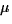m). By quantum well design the properties of the localized ''quantum'' species can be tuned and optimized for applications. Due to their localized nature the above ''quantum'' species cannot carry a current within a single-particle approach and therefore acts as a null-space for the transport. In consequence, their occupation remains fixed forever - which is contradicting to physics.

Interaction as phonon-carrier and carrier-carrier scattering will change this simplified picture and causes kinetical processes for all the species. Among others, carriers can then migrate from one species to another. Above a certain time-scale such processes can be modeled in some approximation in terms of a dynamics which effectively counts the amount of carriers being captured by the quantum wells as well as the amount of carriers escaping from the quantum wells.

M. Baro (Berlin):
Kirkner-Lent-Poisson system
We analyse a Schrödinger-Poisson system on the interval, i.e. the electrostatic potential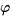is determined by Poisson's equation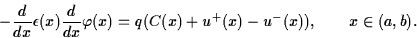The densitiesof electrons and holes are determined by so-called Kirkner-Lent families, i.e. a family of Schrödinger-type operatorswith transparent boundary conditions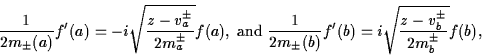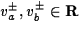,.

This model allows to consider current carrying scattering states. Hence a current coupling with a classical drift diffusion model is possible.

We will show that the Kirkner-Lent-Poisson system always admits a solution and give some a priori estimates.

This is a joint work with P. Degond, H.-Ch. Kaiser, H. Neidhardt, and J. Rehberg.

C. Carstensen (Wien, AT):
Finite Element Approximation of Averaged Quantities in Microstructures
This talk addresses a non-convex minimization problem (M) motivated by variational models (i) for phase transformations in crystal physics -advertised, e.g., by Fonseca, Ball, James; (ii) in optimal design tasks -advertised, e.g., by Kohn; (iii) in micromagnetics -advertised, e.g., by Tartar.

In contrast to convex energy densities, a non-convex density W, typically, leads to non-attainment of the infimal energy in (M) even though W is smooth and satisfies proper growth conditions. The simplest 1D example W(x)=(x2-1)2 due to Bolza serves as a basis to explain the lack of sequentially weak lower semicontinuity and the enforced high oscillations of infimizing sequences called microstructures.

The presentation illustrates those phenomena and their impact on a finite element simulation (Mh): Strong convergence of gradients is impossible! Essentially two relaxation results from state-of-the-art calculus of variations are available to cure the problem. Young measures and (quasi-) convexification are the mathematical key concepts behind the proposed numerical alternatives (Gh) and (Qh). Recent numerical algorithms and convergence results form the center of the presentation. Mathematical difficulties encountered in enforced microstructures include non-quadratic growth conditions as well as a degenerated or even non-convex relaxed energy functional. Comments on remedies to gain strong convergence of gradients, open questions, and future developments conclude the talk.

M. Ehrhardt (Saarbrücken):
Discrete transparent boundary conditions for the Schrödinger equation: Fast calculation, approximation, and stability
This talk is concerned with transparent boundary conditions (TBCs) for the time-dependent Schrödinger equation in one and two dimensions. Discrete TBCs are introduced in the numerical simulations of whole space problems in order to reduce the computational domain to a finite region. Since the discrete TBC for the Schrödinger equation includes a convolution w.r.t. time with a weakly decaying kernel, its numerical evaluation becomes very costly for large-time simulations.

As a remedy we construct approximate TBCs with a kernel having the form of a finite sum-of-exponentials, which can be evaluated in a very efficient recursion. We prove stability of the resulting initial-boundary value scheme, give error estimates for the considered approximation of the boundary condition, and illustrate the efficiency of the proposed method on several examples.

G. Friesecke (Warwick, UK):
Long time dynamics of Fermi-Pasta-Ulam lattices: persistence of coherent modes and recurrence theorem for Fourier spectrum
In joint work with R.L.Pego (Maryland), we have recently obtained a recurrence theorem related to a fundamental question raised by Fermi, Pasta and Ulam in 1947.

F, P and U wondered numerically about the long time behaviour of the Fourier spectrum of a 1D nonlinear atomic chain with Hamiltonian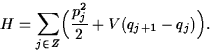Statistical mechanics reasoning suggests that the nonlinearity would promote asymptotically thermalized distribution of energy among the Fourier modes. (For a linear lattice, i.e. a quadratic potential V, the spectral density would be time-independent). But the numerical experiments showed strong recurrence effects, which have remained poorly understood.

Our theorem says that given any energy surface H=E with sufficiently low energy E, and given any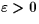, there exists an open set of initial data whose Fourier spectral density is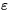-recurrent. This means that after each integer multiple of some recurrence time T, the spectral density has distance at most(in the L1 norm) from the initial density.

Our construction of recurrent regions is related to the presence of solitary wave modes in the lattice. (Much of the literature has instead been viewing the FPU recurrences as KAM type effects. But it seems it has not been possible to turn this idea into a theorem. I will discuss an explanation suggested by E.Wayne.)

K. Gelfert (Dresden):
Langevin model for the slow motion in a deterministic multi-scale system
In this talk we intend to establish a novel approach for the modelling and effective simulation of systems with time-scale separation. For a class of deterministic dynamical systems where the state space variables can be divided into a group of fast and a group of slow variables it will be discussed how a fast chaotic motion can be modelled by suitable stochastic forces. Here, projection techniques, which are well known from non-equilibrium statistical mechanics, are employed to eliminate the fast motion. After an approximation step a Fokker-Planck equation governing the evolution of the density of the slow variables is derived. In this equation the diffusion term is given in terms of correlation properties of the fast motion while the drift term consists of an adiabatic average of the slow motion plus a renormalization by chaotic fluctuations.

J. Giannoulis (Stuttgart):
Young-measure solutions to a generalized Benjamin-Bona-Mahony equation
We are interested in the evolution of macroscopic properties of microscopic structure.

Starting from the viewpoint that microstructure can be modelled by spatial highly oscillatory initial data, in order to obtain its macroscopic information one is tempted to use the limit achieved as the (micro-)period tends to zero, i.e, to use Young measures. Indeed, Young measures maintain the macroscopic properties of oscillations (the simplest examples being the main value, the amplitude etc.), while they fade out the microscopic information.

Since we want to describe the evolution of the macroscopic features of our data, we have to derive a macroscopic evolution equation for Young measures from the given (microscopic) equation, the latter describing the evolution of the oscillations.

This programme will be outlined for the case of a generalization of the BBM equation (an alternative'' to the KdV equation) showing exemplarily the (also numerical) advantages of the application of such an approach.

S. Goedecker (Grenoble, FR):
Wavelets, Plane Waves and Multigrid Methods in the Context of Poisson's Equation
Wavelets, plane waves and multigrid methods share many central ideas. An unified point of view can help us in the construction of more efficient algorithms. In particular it will be shown how Poisson's equation discretized in a wavelet basis can be solved efficiently in a multigrid way and how multigrid algorithms can be improved by incorporating wavelet concepts.

M. Herrmann (Berlin):
Micro-macro transitions in the atomic chain
In this talk we consider micro-macro transitions in an atomic chain whose microscopic dynamics is described by a large system of Newton equations with a nonlinear but convex interaction potential. Starting form the hyperbolic scaling of space and time we discuss the thermodynamical (macroscopic) describtion of the chain for a suitable class of microscopic initial data. In particular, we identify a closure principle that describes the statistical distribution of the atoms for any macroscopic space time point. Using this closure principle we can describe the macroscopic behaviour of the chain by a system of hyperbolic pde's, namely the well known Euler system of thermodynamics. Finally, we study Riemann problems for the atomic chain.

A. Jüngel (Konstanz):
Macroscopic semiconductor modeling and simulation
The ongoing miniaturization of semiconductor devices reached nowadays a length scale at which quantum effects play a dominant role. Therefore, standard models like the classical drift-diffusion equations are physically inaccurate and have to be replaced by models which incorporate the relevant quantum effects. The state of the art in quantum semiconductor modeling ranges from microscopic models, like Schrödinger or Wigner equations, to macroscopic equations, like quantum hydrodynamic models.

This talk gives an overview of various macroscopic quantum models and presents analytical and numerical results on some of these models, in particular the (1) quantum drift-diffusion, (2) quantum hydrodynamic, and (3) viscous quantum hydrodynamic equations.

K. Kirchgässner (Stuttgart):
Travelling waves in a chain of coupled nonlinear oscillators
In a chain of nonlinear oscillators, linearly coupled to their nearest neighbors, all travelling waves of small amplitude are found as solutions of finite dimensional reversible dynamical systems. The coupling constant and the inverse wave speed form the parameter space. The ground state consists of a one-parameter family of periodic waves. It is realized in a certain parameter region containing all cases of light coupling. Beyond the border of this region the complexity of wave-forms increases via a succesion of bifurcations. An appropriate fomulation of this problem will be given, and the basic facts about the reduction to finite dimensionional systems will be indicated. We show the existence of the ground state and discuss the first bifurcation via normal form arguments. Furthermore we show the existence of nanopterons, i.e. localized waves with noncancelling periodic tails at infinity which are exponentially small in the bifurcation parameter. (Joint work with G.Iooss, CMP 211(2000)).

C. Lasser (München):
Molecular dynamics and energy level crossings
The Born-Oppenheimer approximation to quantum-mechanical molecular dynamics locally breaks down in the presence of energy level crossings. There are various types of energy level crossings, each of it associated with an own Landau-Zener formula. An asymptotic analysis of a Schrödinger equation with level crossing can be carried out by taking the solution's Wigner transform and passing to the semiclassical limit; an approach, which has been introduced to this problem class by C. Fermanian and P. Gérard. We will adopt this point of view and discuss some simple model problems with avoided crossing, codimension one and codimension two crossing.

H. Luo (Kassel):
Wavelet approximation of correlated wavefunctions
We suggest an alternative approach for the local treatment of electron corelation based on numerical methods from multiscale analysis. By this we are aming to achieve a better description of the various length- and energy-scales inherently connected with different types of electron correlations. For the first step, we take the local ansatz which corresponds to a coupled electron pair approximation (CEPA), and approximate the correlation part by means of hyperbolic wavelets. We perform diagramatic analysis for the calculation of the matrix elements.

K. Matthies (Berlin):
Atomic-scale localization of high-energy solitary waves on lattices
One-dimensional monatomic lattices with Hamiltonian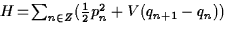are known to carry localized travelling wave solutions, for generic nonlinear potentials V. In this talk we derive the asymptotic profile of these waves in the high-energy limit, for Lennard-Jones type interactions. The limit profile is proved to be a universal, highly discrete, piecewise linear wave concentrated on a single atomic spacing. This shows that dispersionless energy transport in these systems is not confined to the long-wave regime. (Joint work with G. Friesecke, Physica D, to appear)

F. Mehats (Tolouse, FR):
Analysis of a Drift-Diffusion-Schrödinger-Poisson model
(joint work with N. Ben Abdallah and N. Vauchelet)

We present the analysis of a coupled quantum-classical system, modeling the transport of a quasi bidimensional electron gas confined in a nanostructure. The coupling occurs in the momentum variable: the electrons are like point particles in the directions x parallel to the gas (classical transport) while they behave like waves in the transversal direction z (quantum description).

The transport of the gas is described by a 2D Drift-Diffusion equation, governing the evolution of a surfacic density ns. The originality of this system is that the parameters of this equation keep a trace of the quantum confinement in the transversal direction. Indeed, the effective potential which gives the drift current is calculated with the subband model through the resolution of an adiabatic Schrödinger-Poisson system. It takes into account the selfconsistent electric potential generated by the electrons and the quantification of the energy in the z variable. The system can be obtained, at least formally, as the diffusion limit of a Boltzmann-Schrödinger-Poisson system.

Let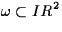be a regular and bounded domain and let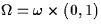. The spatial variables are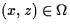.The model studied here is the following coupled system: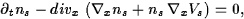(1)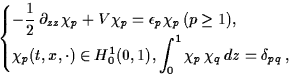(2)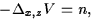(3)
where the unknowns are the surfacic density ns(t,x), the eigen-energies, the eigenfunctions,and the electrostatic potential V(t,x,z). These equations are coupled through the density n and the effective potential Vs, which are defined by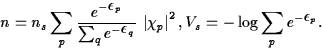(4)
This system (1)-(4) is completed with an initial condition and conservative boundary conditions. Using a fixed-point procedure, we have proved the existence of a unique solution to this nonlinear system.

J. M. Melenk (Leipzig):
Generalized FEM and two-scale regularity for homogenization problems
We present regularity results for the solutions of a class of elliptic boundary value problems with rapidly oscillating, periodic coefficients. At the heart is a detailed analysis of the so-called unit-cell problem. Applications of our results include mesh design principles for the generalized FEM (gFEM). The gFEM introduced by A.-M. Matache and C. Schwab is a projection method with problem-adapted ansatz space that can lead to robust convergence, i.e., the convergence is independent of the coefficients' period. In this talk, we will review the gFEM and elaborate the bearing of our regularity results on it.

A. Mielke (Stuttgart):
Macroscopic dispersive energy transport in harmonic lattices
We study oscillations of an infinite periodic lattice in one or several space dimensions. We consider the atomic distance 1/n as the small microscopic length scale and the aim is to derive macroscopic evolution laws. The simplest model displaying already most features is the one-dimensional chain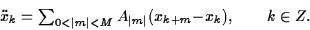From the microscopic dispersion relation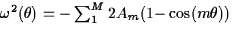we have the exact solutions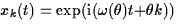. Using Fourier transform yields the general solutions.

Defining the macroscopic functions, with sinc,in the macroscopic variablesand y=z/n we obtain via weak convergence arguments that the associated macroscopic limit equation is the linear wave equationThe microscopic energy distribution distribution is a quadratic functional of the vleocities and the strains. It is shown that the Wigner transform can be used to describe its weak limit. The associated limit foris a measure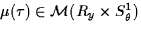which has to satisfy the transport equation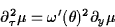in the sense of distributions.

H. Neidhardt (Berlin):
Current coupling of drift -diffusion and Schrödinger-Poisson systems
(joint work with M.Baro, H.-Chr.Kaiser,and J.Rehberg)

Let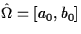be an closed interval on the real axis which contains the closed interval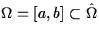. On the semi-intervals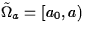and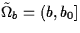, which are called classical zones, one-dimensional drift-diffusion models without generation and recombination are considered while on the closed interval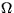, which is called the quantum zone, a description by dissipative Schrödinger-Poisson systems with given densities matrices (steady states) is assumed. Both systems are coupled to a hybrid model as follows:

1.
The boundary coefficients of the dissipative Schrödinger-Poisson systems are determined by the quasi-Fermi levels of the drift-diffusion models in accordance with dissipative Kirkner-Lent models, see talk of M. Baro.
2.
The current densities are constant for the coupled system, in particular, current densities for the classical and quantum zones are equal (current coupling).

It is shown that under these assumptions the hybrid model has always a solution which is in general not unique.

R. Schneider (Chemnitz):
Wavelet subspace splitting
Wavelet compression is a favourable tool for an efficient approximation of singularities. Moreover the hierarchical description admits a hyperbolic cross or sparse grid approximation, reducing the complexity of multidimensional problems. Both can be used for the numerical ab initio solution of many particle Schrödinger equation. Even Kato type singularies are resolved adaptively. However these results are limited and not satisfactory. At this point it becomes necessary to go beyond classical wavelet analysis. The complexity is too high, because the information encoded by the wavelet bases is still redundant. The idea to overcome this deficiency is to split the wavelet spaces itself into several subspaces where only a low dimensional subspace contains the essential information. This may be viewed as a better and more appropriate localization in the frequency domain. In fact, we are constructiong wavelet subspaces with higher order vanishing moments by linear combination of wavelet functions. The resulting basis functions are builting again Riesz bases. We show how to apply this construction to resolve Kato type singularities. At the end we apply the present concept to construct a stable wavelet methods which is exponetially convergent for analytic datas. By the introduction of new basis functions we have enriched our libary of basis functions. A best N-term approximation becomes now a problem of finding best bases. The strategy to find this bases depends on which a priory information is available.

S. Teufel (München):
Effective dynamics in a periodic potential: Peierls substitution and beyond
The dynamics of electrons in a crystal can be described in good approximation by the Schrödinger equation for a single particle with a periodic potential. Typically external magnetic and electric fields are weak compared to the periodic field and thus the external potentials have a slow variation on the scale set by the lattice. As a consequence of this separation of scales the macroscopic dynamics of the electron are governed by an effective Hamiltonian obtained through the famous Peierls substitution''. We present a perturbative scheme which allows not only for a rigorous justification for Peierls substitution'', but also yields corrections to arbitrary order in the small parameter describing the separation of scales.

F. Theil (Warwick, UK):
Surface energies in lattice models
Probably the best known finite scale effects in atomistic systems are surface energies. In a simplistic, two-dimensional model the total energy E associated to the atom positions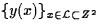is given by the pair sum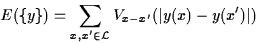where the potential Vx-x' is harmonic if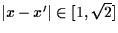and 0 else. This corresponds to a system of mass points coupled via linearly elastic springs. Forwhere R>0 is a scaling parameter and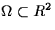is a a continuum domain the minimum energy scales in the following wayThe surface term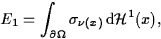where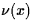is the surface normal at x, encodes information about the relaxation pattern of the atoms close to the surface. We show that in many cases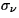can be found by solving 1-periodic cell problems. The result can be described as a Cauchy-Born rule for surfaces.

When the surface relaxation is ignored,depends smoothly on the normal vector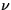except on a closed set of measure 0. The nontrivial relaxation pattern of the atom positions towards the interiour changes the qualitative behaviour ofdramatically. Numerical studies indicate that even for small surface relaxationmight be nowhere smooth in.